# Paper Cutting

In a Competitive exam, Reasoning is one of the most important section. A good score in Reasoning test can lead you to score very good marks in competitive exams. You can achieve a good marks only if you have a depth knowledge of reasoning skills. Basically reasoning is divided into few sections according to logic and type. This Paper Cutting topic is one of them.

"Paper Cutting is a process in which a piece of paper (of any shape) is folded along a certain direction and then cuts are made into it."

Different types of questions covered in this chapter are as follows

1. Selecting Unfolded Pattern of a Folded Punched Piece of Paper
2. Selecting Folded and Punched Pattern Based on Unfolded Pattern

In questions based on paper cutting, one or more question figures are given showing the manner in which a piece of paper is folded, where a dotted line is the reference line along which the paper has to be folded and the arrow indicates the direction of the fold. Then the paper is punched (cut ) from a particular section. Such cuts may be based on varying patterns. These patterns appear on the paper when the paper is unfolded after cutting. The candidates are required to see the question figure(s) carefully and then identify the unfolded paper having correct cutting pattern out of the four answer choices given in the form of figures.

Let us see some figures which are being folded, cut and then unfolded to have a basic idea about the concept of paper cutting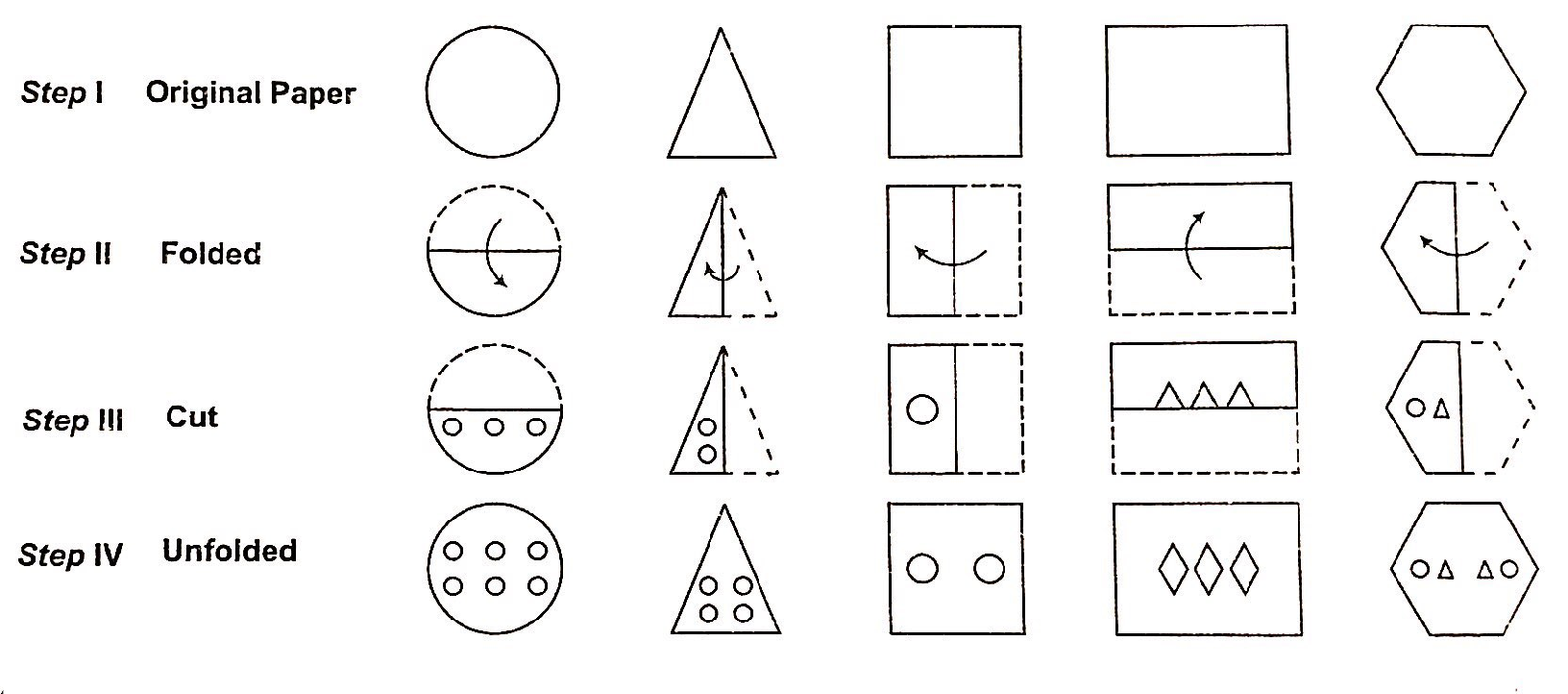Note:

1. The above presentations depict fold punching (cutting). Questions based on paper cutting may involve multiple folds.
2. The cuts that are shown in the above presentation may be in made any form.

Type #1 : Selecting Unfolded Pattern of a Folded Punched Piece of Paper

In this type of questions, the order in which a paper is folded and its punching pattern after folding is depicted through a series of question figures. The objective is to select from among the four answer figures, the one which correctly shows the shape of the paper after it is unfolded.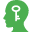Memory Add-ons

When more than one fold is made before punching then virtually try to unfold each fold one by one and predict the complete unfolded pattern.

The following examples will give you a better idea about such type of questions

Example 1: A piece of paper is folded and cut as shown below in the question figures. From the given answer figures, indicate how it will appear when opened.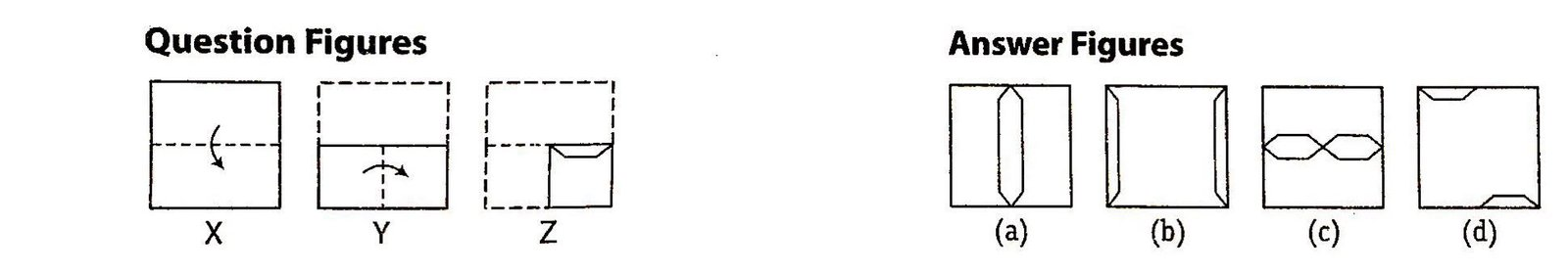Solution: (c)  It is clear that in figure (X), the paper has been folded from the upper half to lower half. In figure (Y), the paper has been folded fro left to right making it one-fourth of the original size. Now, as given in figure (Z), the cut in made in such a way that the design of the cut will appear along the central line of the page, when it is unfolded. Hence, answer figure (c) represents the correct unfolded shape of the paper after the cut.

Example 2 : The three question figures marked X, Y, and Z show the manner in which a paper is folded step by step and then cut. From the answer figures (a),(b),(c) and (d), select the one showing the unfolded pattern of the paper after the cut.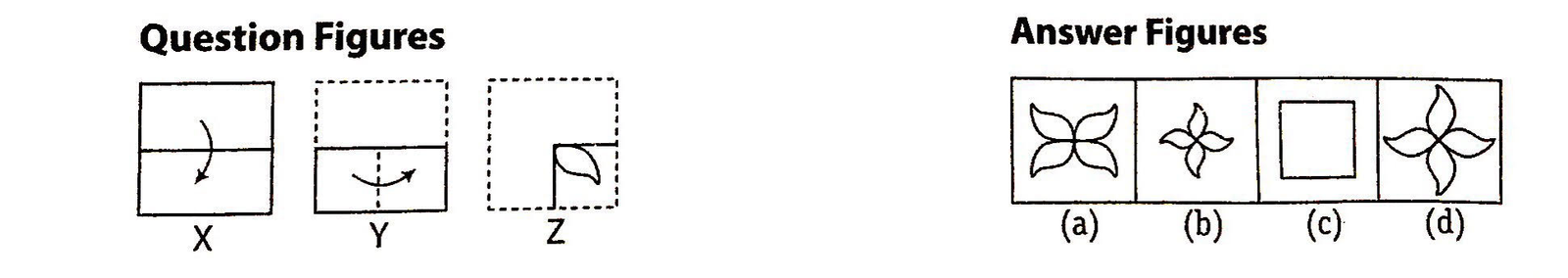Solution: (a)  It is clear that in figure (X), the paper has been folded from the upper half to lower half. In figure (Y), the paper has been folded fro left to right making it one-fourth of the original size. Now, as given in figure (Z), the cut is marked. The design of the cut will appear as in answer figure (a), when it is unfolded. Hence, answer figure (a) represents the correct unfolded shape of the paper after the cut.

Type #2 : Selecting Folded and Punched Pattern Based on Unfolded Pattern

In such type of questions, a question figure is given which represents an unfolded piece of paper with several punches (cuts) made into it along with it, four alternatives representing differently folded and punched paper patterns are also given. The candidates is required to analyse the answer figures to find out the correct folded and punched paper pattern for the given unfolded pattern.

Example 1: A paper sheet is folded in a particular manner and several punches (cuts) are made. When unfolded the paper sheet looks like the question figure (X). From the given options select the one that follows the manner in which the paper is folded and punched.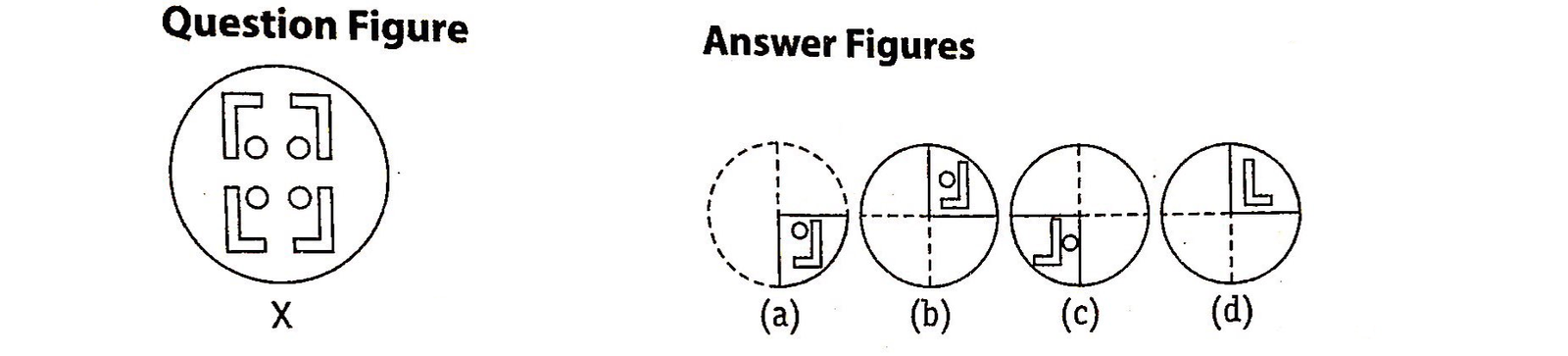Solution: (a) When we fold question figure (X), then it look like answer figure (a).

Example 2: A paper sheet is folded in a particular manner and several punches (cuts) are made. When unfolded the paper sheet looks like the question figure (X). From the given options select the one that follows the manner in which the paper is folded and punched.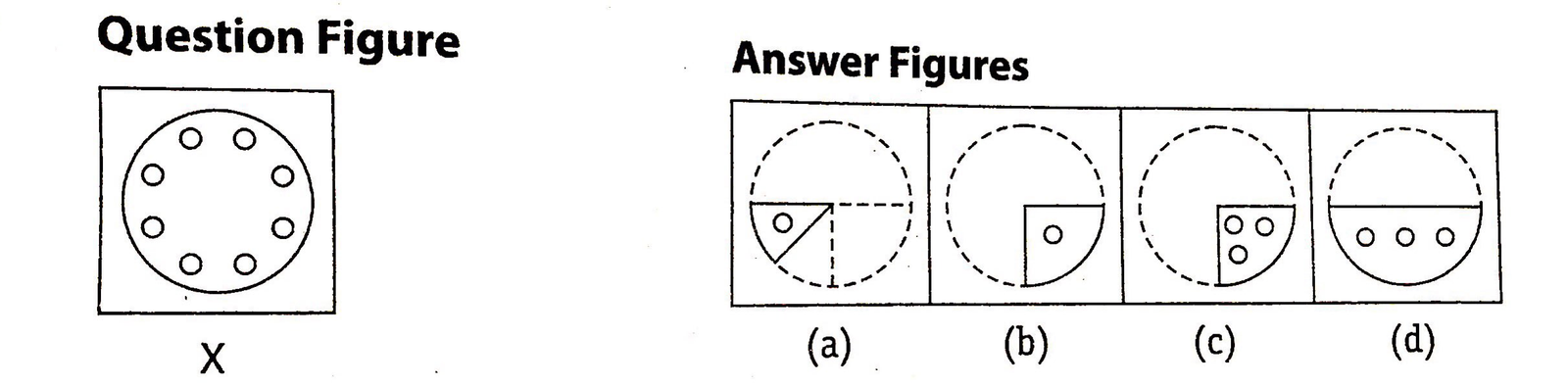Solution: (d) When we fold question figure (X), then it look like answer figure (d).

## TIPS on cracking Non Verbal Reasoning Questions on Paper Cutting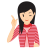Few Important things to Remember

Time is a very important thing in every exams. You should complete your exam within the time frame. But in a competitive exam, question are for testing your calculation ability within a given time frame. They tests, how quickly a student can solve a question paper. This is the reason so many students couldn’t finish their paper within given time. But if you need to solve your government or bank or any other question paper quickly then you should use tips of reasoning and Paper Cutting.

Tip# : For problems on Paper Cutting, imagine the process of folding, cutting and opening, and draw indicative diagrams if required

Question: Choose a figure which would most closely resemble the unfolded form of Figure (Z).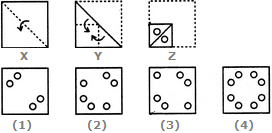Solution: (4) Resemble the unfolded form of Figure (Z), then it look like answer figure (4).